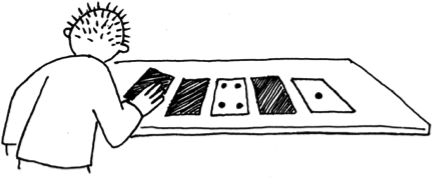Binary Numbers

Count the Dots #

The binary number system plays a central role in how information of all kinds is stored on computers. Understanding binary can lift a lot of the mystery from computers, because at a fundamental level they’re really just machines for flipping binary digits on and off. There are several activities on binary numbers in this document, all simple enough that they can be used to teach the binary system to anyone who can count! Generally children learn the binary system very quickly using this approach, but we find that many adults are also excited when they finally understand what bits and bytes really are.Photos #

An older version of this activity can be downloaded in PDF format here. The content is similar to the current version, but there’s some extra technical information.

If you want to find out more #

• Wikipedia: Computer Numbering Formats,
• Wikipedia: Binary Numbers
• Wikipedia: Finger Binary.
• Wikipedia: Most Significant Bit
• Wikipedia: Logic Gate
• Wikipedia: Powers of Ten
• Other number systems on Wikipedia:
• Decimal: The decimal numeral system (also called base ten or occasionally denary) has ten as its base. Positional decimal systems include a zero and use symbols (called digits) for the ten values (0, 1, 2, 3, 4, 5, 6, 7, 8, and 9) to represent any number, no matter how large or how small.
• Hexadecimal : uses sixteen distinct symbols, most often the symbols 0–9 to represent values zero to nine, and A, B, C, D, E, F (or alternatively a through f) to represent values ten to fifteen.
• Octal: The octal numeral system, or oct for short, is the base-8 number system, and uses the digits 0 to 7. Numerals can be made from binary numerals by grouping consecutive binary digits into groups of three (starting from the right).
• ASCII: The American Standard Code for Information Interchange (acronym: ASCII; pronounced /ˈæski/, ASS-kee) is a character-encoding scheme based on the ordering of the English alphabet. ASCII codes represent text in computers, communications equipment, and other devices that use text.
• Virginia Tech, Dept of Computer Science has a complete module on Number Systems.
• Ron Hale-Evans has a Wiki entry called Binary Numbers System.
• Ken Bigelow has a website Digital Logic that covers most topics related to binary and digital logic.
• Jeremy Falcon has an excellent article on Learning Binary and Hexadecimal.
• Exploring Binary has the following interesting sections on the Powers of 2:
• The Powers of Two: Why are they called powers of two? What is the pattern you see? How is the set described mathematically? What are the set’s components? We will answer those questions in this article.
• 1,073,741,823 Grains of Rice : In the children’s book “One Grain of Rice: A Mathematical Folktale” a girl uses her knowledge of exponential growth to trick a greedy king into turning over his stockpile of rice. Hidden in the story are mathematical concepts related to doubling: powers of two, geometric sequences, geometric series, and exponents. I will analyze the story from this perspective, and then discuss my experience reading it to first and third grade students.
• Exploring Binary Numbers With PARI/GP Calculator: PARI/GP is a sophisticated tool, with several components — yet it’s easy to install and use. I use its command shell in particular, the PARI/GP calculator, or gp for short. I will show you how to use simple gp commands to explore binary numbers.
• Powers Of Two In The Josephus Problem: This formula, you won’t be surprised to hear, has connections to the powers of two and binary numbers. I will discuss my favorite solution, one based on the powers of two.
• Elements of Binary in the NCAA Basketball Tournament: If you’re like me, you also think of powers of two, binary trees, logarithms, laws of exponents, geometric sequences, geometric series, and Bernoulli trials — in short, the elements of binary numbers, binary code, and binary logic.
• Dr. John H. Lienhard has the following interesting articles on the history of different number bases:
• howtoons illustrates counting in binary numbers using cartoons:
• Kerry Redshaw has a website with information on pioneers in the history of computing. The following articles are of interest:
• Hierosolyma Kadathian’s page on Numeric Systems defines number systems, then provides information about binary and the hexadecimal system.
• Math Steps provides a good explanation and teacher resources on Place Values.
• NCETM’s Seconday Magazine has the following articles of interest:
• Focus on…shunting, an article on an application of binary notation in which the challenge is to rearrange railway trucks with as few shunts as possible, provide situations in which a teacher can focus on some particular ways of acting mathematically
• Focus on…perfect shuffles: There are some magic tricks that use pretty elaborate mathematics…and…magicians can perfectly shuffle a deck of cards. Explore the link to Binary numbers in this activity
• Exploring Digital Devices: exploring base 2 numbers
• TI-Basic Developer has a section on Binary, Hexadecimal and Octal Number System which explains these systems and their applications